# Reverse a Linked List in Groups

## Introduction

Linked List has often been one of the hot topics generally asked in Interviews, and reversing a linked list is a very common question. The problem of reverse a linked list in groups is a slight variation to the problem “Reverse a Linked List”.

The question discussed in this blog will cover two important concepts:

2. Traversing a linked list and manipulating pointers

The problem statement is that we are given a linked list containing n nodes. We are also given an integer k which is less than or equal to n. Now the task is to reverse every group of k nodes. If the number of nodes left in the Linked List to be reversed is less than the k, then leave it.

Let’s take an example:
You are given the following linked list with N = 6 nodes and k = 2.Now let us walk through the example:
We have to reverse the set of k nodes and then repeat the process until we reach the end of the Linked List.
So we will reverse pairs 1 and 2, 3 and 4, 5 and 6, respectively. We have 3 sets of nodes having 2 nodes each.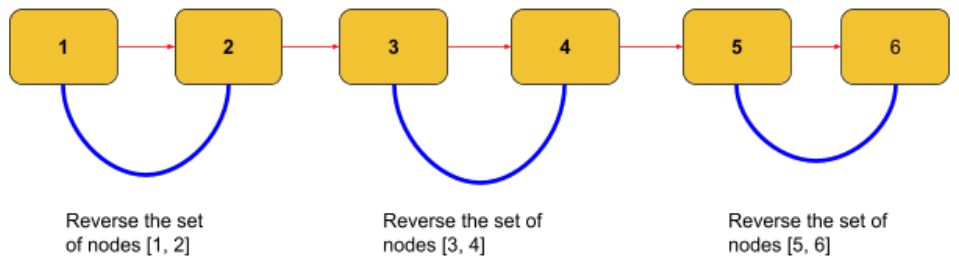On reversing the set of K nodes and reconnecting the Linked List, the output will look like as follows: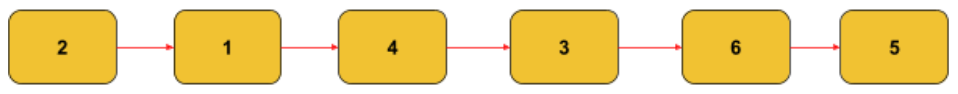Let’s see another example:
You are given the following linked list with N = 5 nodes, and k = 3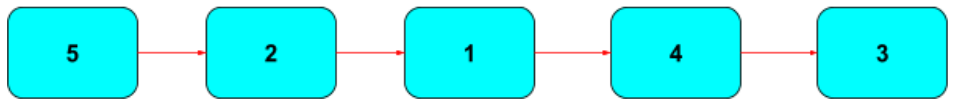Now let us walk through this example:
We have to reverse the set of k nodes and then repeat the process until we reach the end of the Linked List.
So we will reverse 5, 2 and 1, respectively. We have 1 set of nodes having 3 nodes. We will leave the remaining nodes because the next set has less than k nodes.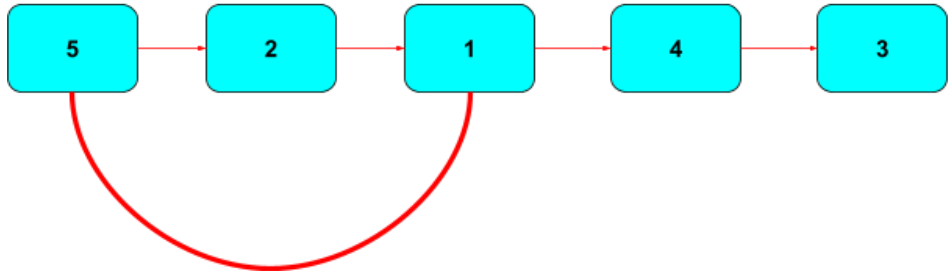On reversing the set of K nodes and reconnecting the Linked List, the output would look like as follows: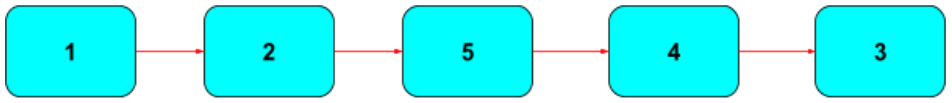## Approach

From the above examples, the approach is pretty intuitive to us.

Let’s look at the approach that comes to our mind first. (Note: we are not talking about thinking of the CODE directly. This is a common issue with all of us these days.)

1. Firstly, we will see if we can find the first K nodes in the Linked List.
1. If we can find k nodes, we will reverse those nodes.
2. If we can’t find it, we can leave the List without reversing it.
2. If step ‘a.’ is executed, we will repeat step ‘a.’.

(Note: the connection of the list is maintained after reversing a set of K nodes so that we don’t lose the nodes).
Now we can think of a PseudoCode.

## PseudoCode

#Assuming there is a function reverse(root) that is used in reversing the linked list.

Algorithm

___________________________________________________________________

procedure reverseListInGroups(root, k):

___________________________________________________________________

1. if k==1 or root is NIL do                               #  base case

2. return root

3. end if

4. countNextKnodes  0           #  to count if we have set of K nodes

5. Head ← root                           # variable to store next set of nodes head

6.        isThereNextNode ← Head.next isn’t null   # to check if we can move further

7.        while isThereNextNode and countNextKnodes < k do

9. isThereNextNode ← Head.next is not null

10.                countNextKnodes  ← countNextKnodes + 1

11. end while

12. If countNextKnodes < k do     #  if count of next nodes is < k, we cannot

13. return root                   # proceed further and return the root

14. end if

15. newRoot ← reverse(root)      # new root after reversing the set of K nodes

16. root.next ←  reverseListInGroups(Head, k)  # call the procedure again since

17. return newRoot     # we are doing repetitive work

18. end procedure

___________________________________________________________________

Source: Meme Creator

Explanation of the pseudocode given above:

In the pseudocode, we have written a recursive algorithm because there is repetitive work being done.

We need to check first if there are k nodes that we can reverse. For that, we keep a count of nodes. Now, if the number of nodes is less than k, we simply return. Otherwise, we proceed further.

We keep track of the next set of k nodes head because of 2 reasons. Firstly, reversing the current set of K nodes would reverse the positions of nodes, and to connect them, we will again need to find the head. Secondly, we need to maintain links of the Linked List, i.e. the chain should not break at any stage.

When we reverse the set of K nodes, the head also changes; hence the current head will be at the kth position in a particular set to which it belongs. Therefore we assign the next of the current root to the root returned by recursive call, mainly because the further recursive calls would also do the same work.

## CODE IN C++

``````//C++ program to find minimum number of swaps
#include <iostream>
using namespace std;

// struct Node for storing nodes
struct Node{
int val;
Node *next;
Node(int data){
this->val = data;
this->next = nullptr;
}
};

// simple iterative function that works by
// reversing front K nodes of a given linked list
// by using the pointer to the root
Node *reverseKnodeLL(Node *root, int k){
Node *curr = root;
Node *prev = nullptr;
Node *next;
while(curr!=nullptr and k>0){
next = curr->next;
curr->next = prev;
prev = curr;
curr = next;
--k;
}
return prev;
}

//function that reverses the given linked list
// in groups of k elements
Node* reverseListInGroups(Node* root, int k) {
if(k == 1)                      // base case
return root;
int countNextKnodes = 0;        // to count if we have set of K nodes
Node *curr = root;              // pointer to current root
Node *next_root = nullptr;      // pointer to store next set of nodes head

// check if we have set of K nodes with us
while(curr!=nullptr and countNextKnodes<k){
curr = curr->next;  // move to the next Node
++countNextKnodes;  // increment count
}

// if set of K nodes is not present then return the root
// no need to reverse it
if(countNextKnodes < k)
return root;

next_root = curr;       // root for the rest of the LL to be reversed.
Node *new_root = reverseKnodeLL(root, k);  // new root after reversing K node set
root->next = reverseListInGroups(next_root,k); //call the function for repetitive work
return new_root;        // return the new root after reversals.
}

// function to print a linked list
void printLL(Node* root){
Node* temp = root;
while(temp){
cout<<temp->val<<" ";
temp = temp->next;
}
cout<<'\n';
}

int main() {
int num_Nodes=5;
int k=3;
// creating a linked List consisting of 5 elements
Node *root = new Node(5);           // add Node 5
root->next = new Node(2);           // add Node 2
root->next->next = new Node(1);     // add Node 1
root->next->next->next = new Node(4); // add Node 4
root->next->next->next->next = new Node(3); // add Node 3
cout<<"The linked list before reversing in groups of K: ";
printLL(root);                      //print original list
root= reverseListInGroups(root, k); // reverse the list in groups of K
cout << "The linked list after reversing in groups of K: ";
printLL(root);                      // print the list after reversing in groups of K
return 0;
}``````

Output

Time Complexity: O(n), where n is the number of elements in the array.
The above algorithm takes O(n) time complexity because we only need to traverse the linked list once and perform a single traversal for the whole linked list.

Space complexity: O(n/k)

We mainly used space in the function for reversing a set of k nodes at a time, so the number of sets at max made is (n/k)+1. Hence, O(n/k) is the number of recursive calls made, and the space used is O(n/k).

Suppose you are in an interview and are asked to write a solution with O(1) space complexity. So let’s discuss with you how we can reverse a linked list in groups using a space-optimized solution.

Now the very first question is, where are we consuming space? (It’s crucial to understand and find the root cause of the problem before directly jumping to the solution.)
We are taking space in the recursive calls we are making. (Fortunately, we have an iterative algorithm of reversing a linked list, and hence we used it in the above code.)

So it’s pretty evident that without making recursive calls and using some variables, if we can manipulate the pointers, we will convert the whole solution into an O(1) space-optimized solution.

## Approach to a Space-optimized Solution

We will traverse and reverse the linked list in multiples of K. So first, we will get the count of the nodes. Now, we can do reversals of sets of K node groups at once and keep doing this until we reach the point when the count of remaining nodes is less than k nodes.

We are not going to change the logic of the recursive approach. The only difference is that now we will be using an iterative approach.
So once we check that there are nodes greater than or equal to K nodes, we reverse the first set of K nodes. And to keep track of the head of the next set of K nodes, we can make any reference or pointer variable that will be storing its address so that we don’t have to traverse again and again.

Then further, we will reverse the k nodes at once. The same process is repeated until the count of the remaining nodes is less than k nodes.

## CODE IN C++(Space Optimized)

``````#include <bits/stdc++.h>
using namespace std;

// struct Node for storing nodes
struct Node{
int val;
Node *next;
Node(int data){
this->val = data;
this->next = nullptr;
}
};

//function that reverses the given linked list
// in groups of k elements
Node* reverseListInGroups(Node* root, int k) {
if(root==nullptr or k<=1)         // Base Case
return root;

//Create a temporary pointer which would be
// pointing to the root of the reversed linked lists
Node *temp = new Node(INT_MAX);

//Point temp->next to the root
temp->next = root;

//Typical pointers needed for reversal
Node *pre=temp;
Node* curr=root;
Node* next=temp;
int countOfTotalNodes=0; // to store count of total number of nodes

while(curr != nullptr){             // run the loop to count all nodes in LL
countOfTotalNodes++;    // increment the count of nodes in LL
curr = curr -> next;    // move to the next node.
}

while(k <= countOfTotalNodes){        // iterate until there are < K nodes remaining
curr = pre->next;
next = curr->next;

for(int i=0; i < k-1; ++i){     // loop to run
curr->next = next->next;   // for reversal
next->next = pre->next;    // of k nodes
pre->next = next;          // Number of iterations are
next = curr->next;         // k-1 because one node can't
}                             // reversed

// decrement the count of remaining elements by k
countOfTotalNodes = countOfTotalNodes - k;

pre = curr;
}

// return the root pointed by next of the temp pointer
return temp->next;
}

// function to print a linked list
void printLL(Node* root){
Node* temp = root;
while(temp){
cout<<temp->val<<" ";
temp = temp->next;
}
cout<<'\n';
}

int main() {
int num_Nodes=5;
int k=3;
// creating a linked List consisting of 5 elements
Node *root = new Node(5);           // add Node 5
root->next = new Node(2);           // add Node 2
root->next->next = new Node(1);     // add Node 1
root->next->next->next = new Node(4); // add Node 4
root->next->next->next->next = new Node(3); // add Node 3
cout<<"The linked list before reversing in groups of K: ";
printLL(root);                      //print original list
root= reverseListInGroups(root, k); // reverse the list in groups of K
cout << "The linked list after reversing in groups of K: ";
printLL(root);                      // print the list after reversing in groups of K
return 0;
}``````

Output

Time Complexity: O(n)
Now one might argue that why is it O(n). So the number of times the outer loop runs is O(n/k), and the internal loop runs for ~ k times whenever it executes.
The total number of iterations is k*O(n/k) ~ O(n).

Space complexity: O(1)
We used three variables to manipulate the pointers of the linked list. Hence no extra auxiliary space is used, and therefore the space complexity becomes O(1).

## FAQs

1. How do you reverse every alternate k node of a linked list?
The problem is similar to the problem: reverse a linked list in groups discussed in this blog. The difference is that we reverse every set of K nodes. But here, we will reverse the alternative set of K nodes.

2. How do you reverse a singly linked list algorithm?
This is a general question of reversing a singly linked list. The basic concept is,  say we have the reverse linked list root whose head is ‘root.next’. And then put the first node at the end of the list and mark the ‘root.next’ as null since root has become the end element. To know more about this question, practice here.

3. Is reversing a linked list Hard?
The answer to this question varies from person to person. But if we consider the difficulty of reversing a linked list, it should be regarded as a medium-level problem. Suppose you try to approach any linked list problem by visualizing how the manipulation of pointers will be conducted using diagrams. In that case, the difficulty of the linked list problem decreases and becomes easier for you to solve.

## Key Takeaways

This article taught us how to reverse a linked list in groups of K nodes in the C++ programming language. We discussed their implementation using the recursive method using illustrations, through a pseudoCode and then using a proper code (the general way one should practice linked lists).

We hope you understood the critical techniques and ways to generally solve the Linked List problems